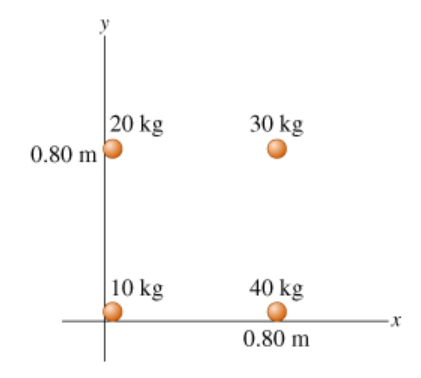# Problem: Find the x coordinate x_cm of the center of mass of the system of particles shown in the figure.

###### FREE Expert Solution

X-coordinate of the center of mass:

$\overline{){{\mathbf{x}}}_{\mathbf{c}\mathbf{m}}{\mathbf{=}}\frac{{\mathbf{m}}_{\mathbf{1}}{\mathbf{x}}_{\mathbf{1}}\mathbf{+}{\mathbf{m}}_{\mathbf{2}}{\mathbf{x}}_{\mathbf{2}}\mathbf{+}\mathbf{.}\mathbf{.}\mathbf{.}\mathbf{+}{\mathbf{m}}_{\mathbf{n}}{\mathbf{x}}_{\mathbf{n}}}{{\mathbf{m}}_{\mathbf{1}}\mathbf{+}{\mathbf{m}}_{\mathbf{2}}\mathbf{+}\mathbf{.}\mathbf{.}\mathbf{.}\mathbf{+}{\mathbf{m}}_{\mathbf{n}}}}$###### Problem Details

Find the x coordinate x_cm of the center of mass of the system of particles shown in the figure.